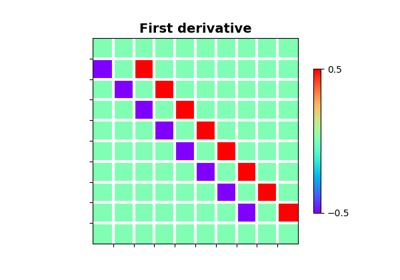# pylops.FirstDirectionalDerivative#

class pylops.FirstDirectionalDerivative(dims, v, sampling=1, edge=False, kind='centered', dtype='float64', name='F')[source]#

First Directional derivative.

Apply a directional derivative operator to a multi-dimensional array along either a single common axis or different axes for each point of the array.

Note

At least 2 dimensions are required, consider using pylops.FirstDerivative for 1d arrays.

Parameters
dimstuple

Number of samples for each dimension.

vnp.ndarray, optional

Single direction (array of size $$n_\text{dims}$$) or group of directions (array of size $$[n_\text{dims} \times n_{d_0} \times ... \times n_{d_{n_\text{dims}}}]$$)

samplingtuple, optional

Sampling steps for each direction.

edgebool, optional

Use reduced order derivative at edges (True) or ignore them (False).

kindstr, optional

Derivative kind (forward, centered, or backward).

dtypestr, optional

Type of elements in input array.

Notes

The FirstDirectionalDerivative applies a first-order derivative to a multi-dimensional array along the direction defined by the unitary vector $$\mathbf{v}$$:

$df_\mathbf{v} = \nabla f \mathbf{v}$

or along the directions defined by the unitary vectors $$\mathbf{v}(x, y)$$:

$df_\mathbf{v}(x,y) = \nabla f(x,y) \mathbf{v}(x,y)$

where we have here considered the 2-dimensional case.

This operator can be easily implemented as the concatenation of the pylops.Gradient operator and the pylops.Diagonal operator with $$\mathbf{v}$$ along the main diagonal.

Methods

 __init__(dims, v[, sampling, edge, kind, ...]) adjoint() apply_columns(cols) Apply subset of columns of operator cond([uselobpcg]) Condition number of linear operator. conj() Complex conjugate operator div(y[, niter, densesolver]) Solve the linear problem $$\mathbf{y}=\mathbf{A}\mathbf{x}$$. dot(x) Matrix-matrix or matrix-vector multiplication. eigs([neigs, symmetric, niter, uselobpcg]) Most significant eigenvalues of linear operator. matmat(X) Matrix-matrix multiplication. matvec(x) Matrix-vector multiplication. reset_count() Reset counters rmatmat(X) Matrix-matrix multiplication. rmatvec(x) Adjoint matrix-vector multiplication. todense([backend]) Return dense matrix. toimag([forw, adj]) Imag operator toreal([forw, adj]) Real operator tosparse() Return sparse matrix. trace([neval, method, backend]) Trace of linear operator. transpose()

## Examples using pylops.FirstDirectionalDerivative#Derivatives

Derivatives